• Posts tagged "假设检验"

# Blog Archives

## 用R语言解读统计检验-F检验

R的极客理想系列文章，涵盖了R的思想，使用，工具，创新等的一系列要点，以我个人的学习和体验去诠释R的强大。

R语言作为统计学一门语言，一直在小众领域闪耀着光芒。直到大数据的爆发，R语言变成了一门炙手可热的数据分析的利器。随着越来越多的工程背景的人的加入，R语言的社区在迅速扩大成长。现在已不仅仅是统计领域，教育，银行，电商，互联网….都在使用R语言。

• 张丹(Conan), 程序员/Quant: Java,R,Nodejs
• blog: http://blog.fens.me
• email: bsspirit@gmail.com

http://blog.fens.me/r-test-f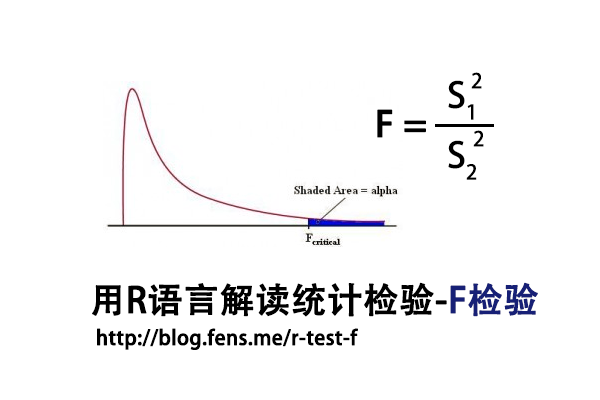1. F检验介绍
2. 数据集
3. F检验实现

## 1. F检验介绍

F检验法(F-test)，初期叫方差比率检验(Variance Ratio)，又叫联合假设检验(Joint Hypotheses Test)，是英国统计学家Fisher提出的，主要通过比较两组数据的方差，以确定他们的密度是否有显著性差异。至于两组数据之间是否存在系统误差，则在进行F检验并确定它们的密度没有显著性差异之后，再进行T检验

F检验，是一种在零假设(H0)之下，统计值服从F-分布的检验。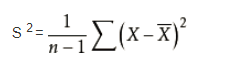F统计量计算公式：

• F:统计量，根据自由度查表，当F值小于查表值时没有显著差异，当F值大于等于查表值时有显著差异
• S1:样本1的标准差
• S2:样本2的标准差
• 分子自由度: df=分子的数量-1
• 分母自由度: df=分母的数量-1

T检验和F检验对比
T检验用来检测数据的准确度(系统误差)，F检验用来检测数据的精密度(偶然误差)。在定量分析过程中，常遇到两种情况：一种是样本测量的平均值与真值不一致；另一种是两组测量的平均值不一致。

T检验是检查两组均值的差异，而F检验是检查多组均值之间的差异。

## 2. 数据集

F检验，对于数据有比较严格的要求，所以我们需要先找到一个合适的数据集，作为测试数据集。我发现了R语言自带的一个数据集ToothGrowth，是很好的测试数据集，本文接下来的内容，将以这个数据集进行测试，来介绍F检验。

• Win10 64bit
• R: 3.4.2 x86_64-w64-mingw32/x64 b4bit

• len列，为牙齿长度
• supp列，为注射方法
• dose列，为注射剂量

``````
len supp dose
1   4.2   VC  0.5
2  11.5   VC  0.5
3   7.3   VC  0.5
4   5.8   VC  0.5
5   6.4   VC  0.5
6  10.0   VC  0.5
7  11.2   VC  0.5
8  11.2   VC  0.5
9   5.2   VC  0.5
10  7.0   VC  0.5
``````

F检验对于数据的正态性非常敏感，我们需要先对选定数据集进行进行正态分布检验。使用Shapiro-Will作为正态分布检验的方法，原假设H0:样本符合正态分布。

``````
# 按不同的处理方法，进行分组
> len_VC<-ToothGrowth\$len[which(ToothGrowth\$supp=='VC')]
> len_OJ<-ToothGrowth\$len[which(ToothGrowth\$supp=='OJ')]

# 正态分布检验
> shapiro.test(len_VC)

Shapiro-Wilk normality test

data:  len_VC
W = 0.96567, p-value = 0.4284

# 正态分布检验
> shapiro.test(len_OJ)

Shapiro-Wilk normality test

data:  len_OJ
W = 0.91784, p-value = 0.02359
``````

``````
> coplot(len ~ dose | supp, data = ToothGrowth, panel = panel.smooth,
xlab = "ToothGrowth data: length vs dose, given type of supplement")
``````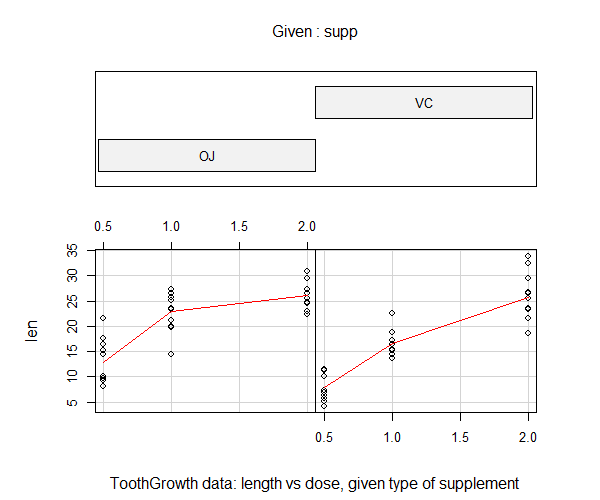## 3. F检验实现

3.1 随机数进行F检验

``````
# 生成随机数
> set.seed(1)
> x <- rnorm(50, mean = 0, sd = 2)
> y <- rnorm(30, mean = 1, sd = 1)

# 进行F检验
> var.test(x, y)

F test to compare two variances

data:  x and y
F = 2.6522, num df = 49, denom df = 29, p-value
= 0.006232
alternative hypothesis: true ratio of variances is not equal to 1
95 percent confidence interval:
1.332510 4.989832
sample estimates:
ratio of variances
2.652168
``````

• H0:原假设2组样本的方差，无显著差异
• F统计量：2.6522
• num df，分子自由度，50-1=49
• denom df，分每自由度，30-1=29
• p-value值：0.006232
• 95 percent confidence interval：95%的置信区间
• ratio of variances：方差比率2.652168

3.2 ToothGrowth进行F检验

``````
> var.test(len_VC,len_OJ)

F test to compare two variances

data:  len_VC and len_OJ
F = 1.5659, num df = 29, denom df = 29, p-value
= 0.2331
alternative hypothesis: true ratio of variances is not equal to 1
95 percent confidence interval:
0.745331 3.290028
sample estimates:
ratio of variances
1.565937
``````

``````
# 手动计算T值
> Xn<-length(len_VC)
> Yn<-length(len_OJ)
> Xm<-mean(len_VC)
> Ym<-mean(len_OJ)

# 计算两组样本的偏方差
> fx<-sum((len_VC-Xm)^2)/(Xn-1)
> fy<-sum((len_OJ-Ym)^2)/(Yn-1)

# 计算F值
> fx/fy
 1.565937

# 手动计算P值，双边检验
> p_value<-pf(f_stat,Yn-1,Xn-1)
> p_value<-2*min(p_value, 1 - p_value);p_value
 0.2331433
``````

http://blog.fens.me/r-test-f## 用R语言解读统计检验-T检验

R的极客理想系列文章，涵盖了R的思想，使用，工具，创新等的一系列要点，以我个人的学习和体验去诠释R的强大。

R语言作为统计学一门语言，一直在小众领域闪耀着光芒。直到大数据的爆发，R语言变成了一门炙手可热的数据分析的利器。随着越来越多的工程背景的人的加入，R语言的社区在迅速扩大成长。现在已不仅仅是统计领域，教育，银行，电商，互联网….都在使用R语言。

• 张丹(Conan), 程序员/Quant: Java,R,Nodejs
• blog: http://blog.fens.me
• email: bsspirit@gmail.com

http://blog.fens.me/r-test-t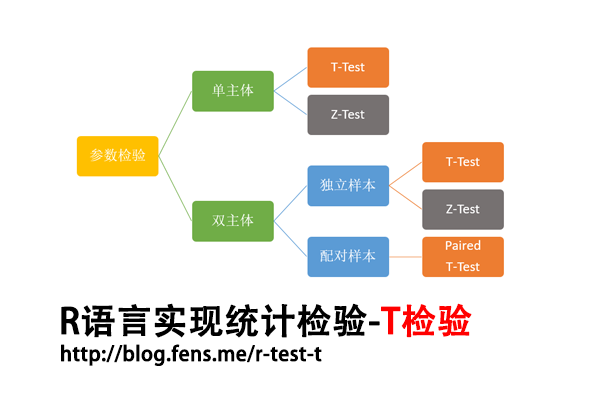1. T检验介绍
2. 数据集
3. 单总体T检验
4. 双总体T检验

## 1. T检验介绍

T检验，也称 student t检验（Student’s t test），是戈斯特为了观测酿酒质量发明的。戈斯特于1908年在Biometrika上公布T检验，但因其老板认为其为商业机密而被迫使用笔名（学生）。

T检验，是用于检验两个小样本的平均值差异程度的检验方法，样本量在3-30个左右，要求样本为正态分布，但总体标准差可未知。T检验，是用T分布理论来推断差异发生的概率，从而判定两个样本平均值的差异是否显著。T检验可分为单总体T检验，双总体非配对T检验，和双总体配对T检验。下面将分别进行介绍。

## 2. 数据集

T检验，对于数据有比较严格的要求，所以我们需要先找到一个合适的数据集，作为测试数据集。我发现了R语言自带的一个数据集sleep，是很好的测试数据集，本文接下来的内容，将以这个数据集进行测试，来介绍T检验。

• Win10 64bit
• R: 3.4.2 x86_64-w64-mingw32/x64 b4bit

• extra列，为睡眠时间的变化
• group列，为药物编号
• ID列，为患者编号

``````
> data(sleep)
> sleep
extra group ID
1    0.7     1  1
2   -1.6     1  2
3   -0.2     1  3
4   -1.2     1  4
5   -0.1     1  5
6    3.4     1  6
7    3.7     1  7
8    0.8     1  8
9    0.0     1  9
10   2.0     1 10
11   1.9     2  1
12   0.8     2  2
13   1.1     2  3
14   0.1     2  4
15  -0.1     2  5
16   4.4     2  6
17   5.5     2  7
18   1.6     2  8
19   4.6     2  9
20   3.4     2 10
``````

Shapiro-Wilk正态分布检验: 用来检验是否数据符合正态分布，类似于线性回归的方法一样，是检验其于回归曲线的残差。该方法推荐在样本量很小的时候使用，样本在3到5000之间。该检验原假设为H0:数据集符合正态分布，统计量为W。统计量W最大值是1，越接近1，表示样本与正态分布匹配。p值，当p-value小于显著性水平α(0.05)，则拒绝H0。

``````
> shapiro.test(sleep\$extra) # 总体正态分布

Shapiro-Wilk normality test

data:  sleep\$extra
W = 0.94607, p-value = 0.3114
``````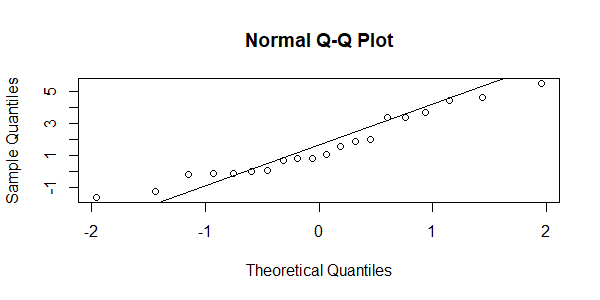## 3. 单总体的T检验

1. 已知总体均值Um
2. 可得到样本均值Xm，及该样本标准误差Xs
3. 样本来自正态或近似正态总体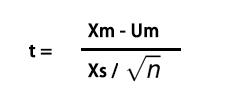• Xm：样本均值
• Um：总体均值
• Xs：样本标准差
• n：样本个数
• 自由度：df=n-1

``````
#  总体均值
> Um<-mean(sleep\$extra)

# 取group=1的样本组
> g1<-sleep\$extra[which(sleep\$group==1)]

# 计算样本均值，方差，数量
> Xm<-mean(g1)
> Xs<-sd(g1)
> n<-length(g1)

# 进行T检验
> t.test(g1,mu=Um)

One Sample t-test

data:  g1
t = -1.3964, df = 9, p-value = 0.1961
alternative hypothesis: true mean is not equal to 1.54
95 percent confidence interval:
-0.5297804  2.0297804
sample estimates:
mean of x
0.75

``````

• H0:原假设总体和样本均值无显著差异
• t统计量：-1.3964
• df，自由度，10-1=9
• p-value值：0.1961
• 95 percent confidence interval：95%的置信区间
• mean：样本均值0.75

``````
# 手动计算T值
> t_stat<-(Xm-Um) / (Xs/(sqrt(n)));t_stat
 -1.396415

# 手动计算P值
> p_value<-2*pt(-abs(t_stat),df=n-1);p_value
 0.1960699
``````

## 4. 双总体T检验

4.1 配对T检验

• 两种同质样本，分别接受两种不同的处理，如性别、年龄、体重、病情程度相同进行配对。
• 同一样本或同一样本的两个部分，分别接受两种不同的处理。
• 同一样本自身对比，把同一组样本处理前后的结果进行比较。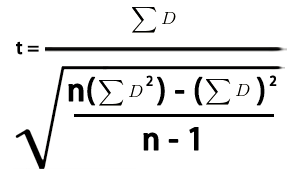• D：两个样本差值
• εD：求和
• ε(D^2)：平方和
• n：样本个数
• 自由度：df=n-1

``````
# 配对T检验
> t.test(extra ~ group, data = sleep, paired = TRUE)

Paired t-test

data:  extra by group
t = -4.0621, df = 9, p-value = 0.002833
alternative hypothesis: true difference in means is not equal to 0
95 percent confidence interval:
-2.4598858 -0.7001142
sample estimates:
mean of the differences
-1.58
``````

``````
# 生成数据集
> g1<-sleep\$extra[which(sleep\$group==1)]
> g2<-sleep\$extra[which(sleep\$group==2)]

# 配对T检验，与上面的计算结果是一致的
> t.test(g1,g2,paired=TRUE)

Paired t-test

data:  g1 and g2
t = -4.0621, df = 9, p-value = 0.002833
alternative hypothesis: true difference in means is not equal to 0
95 percent confidence interval:
-2.4598858 -0.7001142
sample estimates:
mean of the differences
-1.58

# 动手计算
> n1<-length(g1)
> n2<-length(g2)
> D<-g1-g2
> Ds<-sum(D)

# 计算T值
> Ds/sqrt((n1*sum(D^2)-sum(D)^2)/(n1-1))
 -4.062128
``````

4.2 非配对T检验

• n1 和n2 为两样本容量
• Xm1和Xm2为两样本均值
• Xs1和Xs2为两样本的标准差

``````
> t.test(extra ~ group, data = sleep)

Welch Two Sample t-test

data:  extra by group
t = -1.8608, df = 17.776, p-value = 0.07939
alternative hypothesis: true difference in means is not equal to 0
95 percent confidence interval:
-3.3654832  0.2054832
sample estimates:
mean in group 1 mean in group 2
0.75            2.33
``````

``````
# 计算数量，均值，标准差
> n1<-length(g1)
> n2<-length(g2)
> Xm1<-mean(g1)
> Xm2<-mean(g2)
> Xs1<-sd(g1)
> Xs2<-sd(g2)

# 计算T值
> (Xm1-Xm2)/sqrt((Xs1^2/n1)+(Xs2^2/n2))
 -1.860813
``````

``````
> boxplot(extra~group,data=sleep)
``````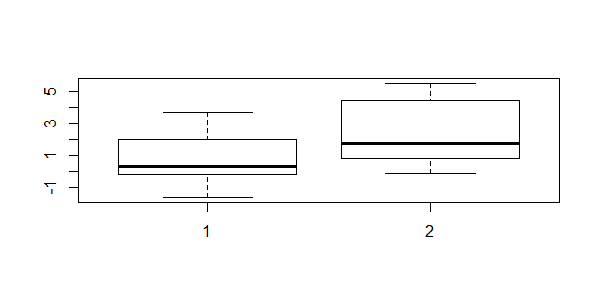http://blog.fens.me/r-test-t